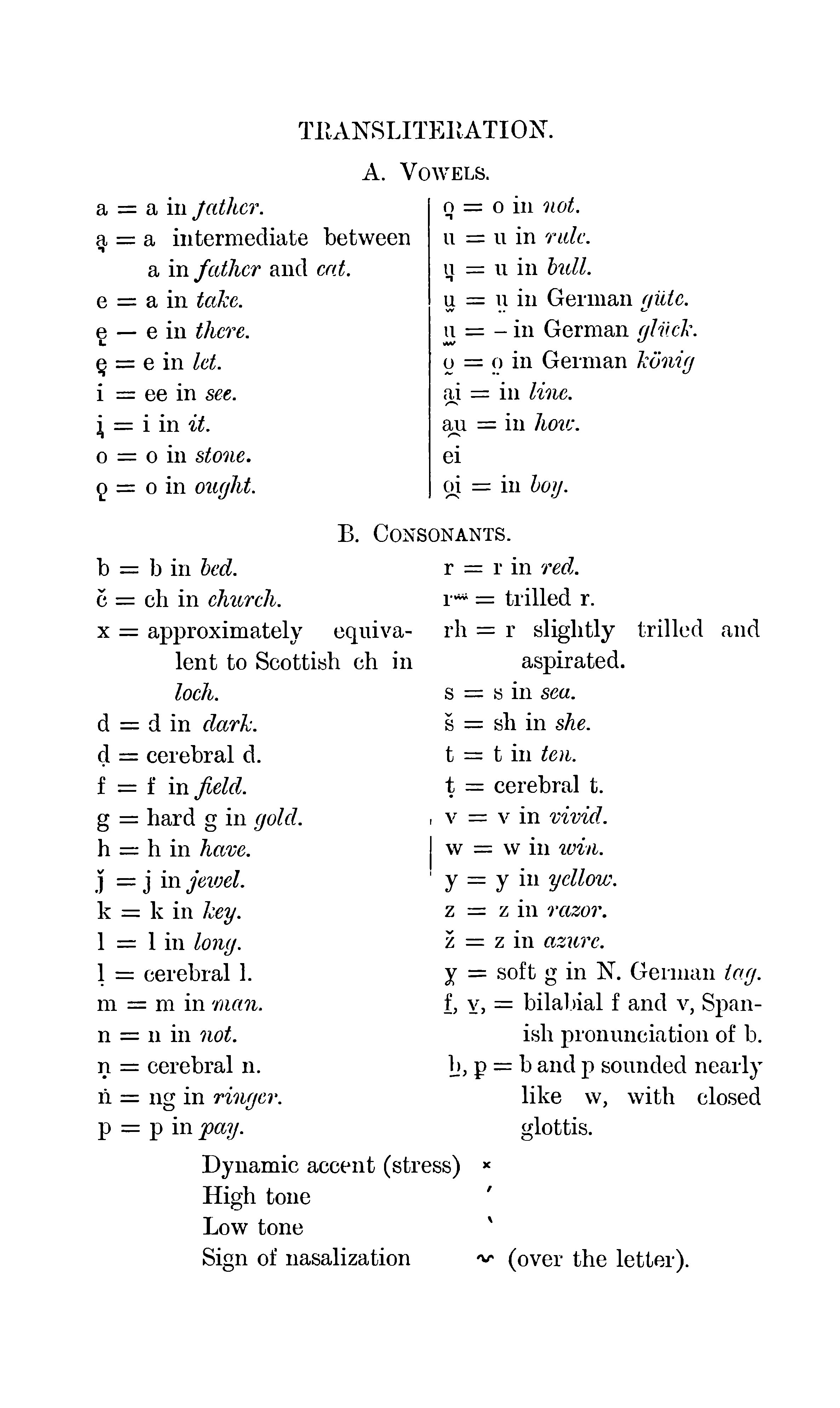# Thomas' Transliteration Table

The characters č, ǰ, u̲, ṳ, o̤, o̰, ḷ, ṇ, ṭ, γ, f, v, b, p and r"" (thrilled r) in the transliteration table do not appear in the dictionary nor in the corpus. The characters ḍ, š and ž appear each two times and the character u̫ one time in the dictionary.

## Scan of the table## Digitized table

TRANSLITERATION.

A. Vowels.

 a = a in father. o͉ = o in not. a͉ = a intermediate between a in father and cat. u = u in ride. u͉ = u in bull. e = a in take. u̫ = ṳ in German güte. ę in there. u̲ = - in German glück. e͉ = e in let. o̰ = o̤ in German könig. i = ee in see. a̯i = in line. i͉ = i in it. a̯u = in how. o = o in stone. ei ǫ = o in ought. o̯i= in boy.

B. Consonants.

 b = b in bed. r = r in red. č = ch in church. r"" = trilled r. x = approximately equiva- lent to Scottish ch in loch. rh = r slightly trilled and aspirated. s = s in sea. d = d in dark. š = sh in she. ḍ = cerebral d. t = t in ten. f = f in field. ṭ = cerebral t. g = hard g in gold. v = v in vivid. h = h in have. w = w in win. ǰ = j in jewel. y = y in yellow. k = k in key. z = z in razor. l = l in long. ž = z in azure. ḷ = cerebral l. ɣ = soft g in N. German tag. m = m in man. f, v, = bilabial f and v, Span- ish pronunciation of b. n = n in not. ṇ = cerebral n. b,p = b and p sounded nearly like w, with closed glottis. ṅ = ng in ringer. p = p in pay.

Dynamic accent (stress) x

High tone   ́

Low tone `

Sign of nasalization ~ (over the letter).